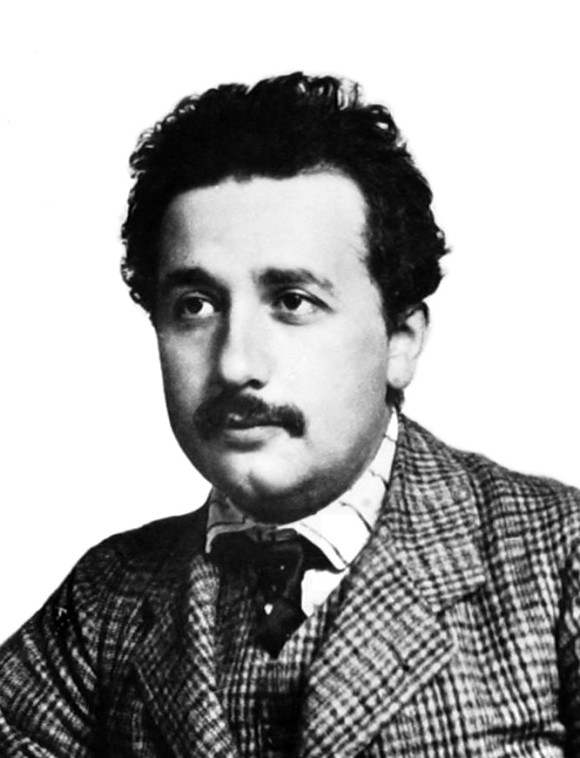Human World

# Today in science: Albert Einstein and E=mc2

September 27, 1905. On this date, while he was employed at a patent office, Albert Einstein published a paper titled “Does the Inertia of a Body Depend Upon Its Energy-Content?” It was the last of four papers he submitted that year to the journal Annalen der Physik. The first explained the photoelectric effect, the second offered experimental proof of the existence of atoms, and the third introduced the theory of special relativity. In the fourth paper, Einstein explained the relationship between energy and mass. That is, E=mc2.

What does it mean? It means that, from the standpoint of physics, energy and mass are interchangeable. In the equation:

E is energy
m is mass
c is the speed of light

In other words, energy = mass x the speed of light squared.

It sounds simple, and its simplicity does belie the genius required of Einstein to express it so elegantly. Mass and energy are interchangeable. Plus, a small amount of mass can equal a large amount of energy; after all, the speed of light is a huge number (186,000 miles per second or 300,000 km/s), and, in Einstein’s famous equation, that huge number is squared. So tiny mass can equal big energy.

E=mc2 explains why the sun and other stars shine. In their interiors, atoms (mass) fuse together, creating the tremendous energy of the sun as described by Einstein’s famous equation.Albert Einstein in 1905, his “miracle year.” Image via Wikimedia Commons.

It’s also why, for example, scientists were able to learn how to build a single bomb that could wipe out a city, such as the atomic bombs that destroyed the Japanese cities of Hiroshima and Nagasaki at the end of World War II.

These early atomic bombs worked due to nuclear fission, not fusion, but they worked on the principle that a tiny amount of mass could be converted to a large amount of energy, as described by Einstein.

Interestingly, the equation E=mc2 does not appear in “Does the Inertia of a Body Depend Upon Its Energy-Content?” That’s because Einstein used V to mean the speed of light in a vacuum and L to mean the energy lost by a body in the form of radiation.

E=mc2 was not originally written as a formula but as a sentence in German that meant:

…if a body gives off the energy L in the form of radiation, its mass diminishes by L/V2.

Einstein’s 1905 paper describing the interchangeable aspect of mass and energy was one of four papers he published during what’s now called his Annus mirabilis or miracle year.

These four articles forever changed our human perception of mass, energy, space and time.Our sun, as seen with an X-ray telescope, showing the corona, the glowing million degree plasma that surrounds the sun. The sun’s energy is produced in its interior, via thermonuclear fusion. That is, mass is converted to energy in a way described by Albert Einstein’s famous equation, E=mc2. Image via Yohkoh satellite.

Bottom line: On September 27, 1905, Albert Einstein published “Does the Inertia of a Body Depend Upon Its Energy Content?” in the journal Annalen der Physik. In it, he described the interchangeable nature of mass and energy, or E=mc2.

Posted
September 27, 2018
in
Human World